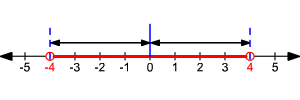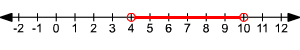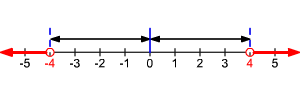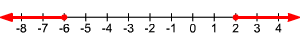# Absolute Value Inequalities

An absolute value inequality is an inequality that has an absolute value sign with a variable inside.

## Absolute Value Inequalities ( $<$ ):

The inequality $|\text{\hspace{0.17em}}x\text{\hspace{0.17em}}|<4$ means that the distance between $x$ and $0$ is less than $4$ .So, $x>-4$ AND $x<4$ . The solution set is $\left\{x\text{\hspace{0.17em}}|\text{\hspace{0.17em}}-4 .

When solving absolute value inequalities, there are two cases to consider.

Case $1$ : The expression inside the absolute value symbols is positive.

Case $2$ : The expression inside the absolute value symbols is negative.

The solution is the intersection of the solutions of these two cases.

In other words, for any real numbers $a$ and $b$ , if $|\text{\hspace{0.17em}}a\text{\hspace{0.17em}}| , then $a AND $a>-b$ .

Example 1 :

Solve and graph.

$|\text{\hspace{0.17em}}x-7\text{\hspace{0.17em}}|<3$

To solve this sort of inequality, we need to break it into a compound inequality .

$x-7<3$ and $x-7>-3$ .

$-3

Add $7$ to each expression.

$\begin{array}{l}-3+7

The graph looks like this:## Absolute Value Inequalities ( $>$ ):

The inequality $|x|>4$ means that the distance between $x$ and $0$ is greater than $4$ .So, $x<-4$ OR $x>4$ . The solution set is .

When solving absolute value inequalities, there are two cases to consider.

Case $1$ : The expression inside the absolute value symbols is positive.

Case $2$ : The expression inside the absolute value symbols is negative.

In other words, for any real numbers $a$ and $b$ , if $|\text{\hspace{0.17em}}a\text{\hspace{0.17em}}|>b$ , then $a>b$ AND $a<-b$ .

Example 2 :

Solve and graph.

$|\text{\hspace{0.17em}}x+2\text{\hspace{0.17em}}|\ge 4$

Split into two inequalities.

Subtract $2$ from each sides of each inequality.

The graph looks like this: